## How to do exponential calculation to a range of cells in Excel?

In Excel, addition, subtraction, multiplication and division is the basic calculation, maybe you can quickly and easily apply them. But sometimes, you will need to do exponential calculation to a range of cells, how can you apply exponential calculation in Excel?

Apply exponential calculation to a range of cells with Power function

Apply exponential calculation to a range of cells with the symbol ^

Apply exponential calculation to a range of cells with Kutools for Excel

####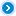Apply exponential calculation to a range of cells with Power function###### Save 50% of your time, and reduce thousands of mouse clicks for you every day!

In Excel, the Power function returns the result of a number raised to a given power.

The syntax for the Power function is: Power (number, power), number is a base number, power is the exponent used to raise the base number to.

For example, Power (10, 2), the number 10 is the base and the number 2 is the exponent. The calculating result is 100.

Now, I have a range numbers (A1:A15), and I want to get these numbers of 3 power.

You can use this function with the following steps:

1. In adjacent blank cell C1, enter this formula: =Power (A1, 3), see screenshot: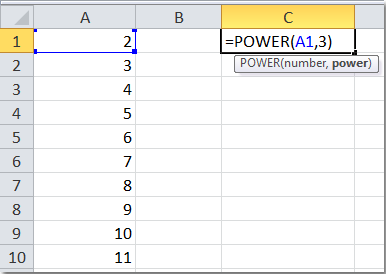2. Then tap Enter key, and select cell C1, then drag the fill handle over to C10. You will get the following results: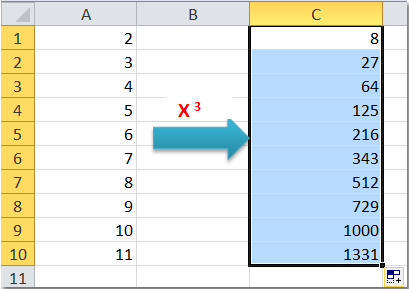3. As they are formulas, when you need copy them to other cells, please paste as values.

####Apply exponential calculation to a range of cells with the symbol ^

As we can find the relevant +, -, *, / symbol on the keyboard, also we can use the symbol ^ to apply the exponential calculations. Such as 10^2, it stands for 10 to the power of 2. And the calculating result is 100. So we can use this way as follows:

1. In adjacent blank cell C1, enter this formula: = A1^3, see screenshot: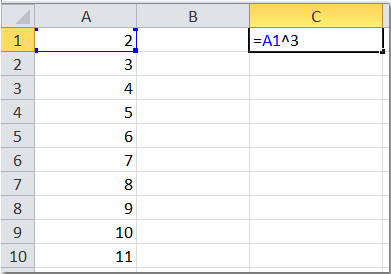2. Then tap Enter key, and select cell C1, then drag the fill handle over to C10. You will get the following results:3. As they are formulas, when you need copy them to other cells, please paste as values.

####Apply exponential calculation to a range of cells with Kutools for Excel

If you are an Excel novice, neither knowing the Power function nor applying the symbol ^, what else method can solve this task?

The Operation Tools of Kutools for Excel can help you to solve this problem quickly and easily.

Kutools for Excel: with more than 300 handy Excel add-ins, free to try with no limitation in 30 days. Get it Now

After installing Kutools for Excel, Please do as follows:

1. Highlight the range you want to do the exponential calculation.

2. Click Kutools > More > Operation Tools, see screenshot: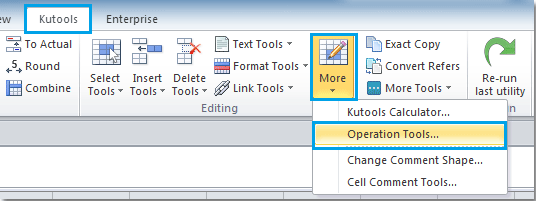3. In the Operation Tools dialog box, select Exponentiation from Operation, and insert 3 in the Operand box, and you can see the results from the Preview Pane. See screenshot: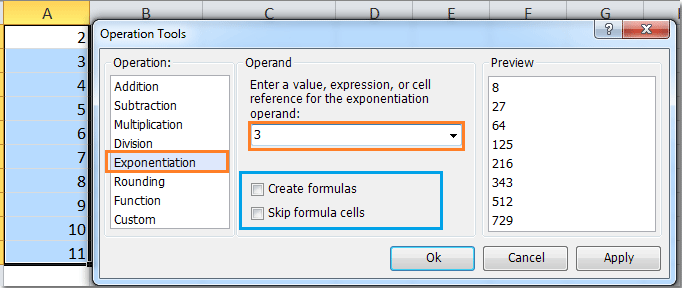4. Then click OK or Apply, You will get the results of these numbers’3 power immediately.

Note: If you want to create formulas as well, you can check Create formulas option. If the selected cells include formulas, and you don’t want to do the exponential calculation to the calculated results of formulas, please check Skip formula cells option.

Related articles:

### Best Office Productivity Tools

Supercharge Your Spreadsheets： Experience Efficiency Like Never Before with Kutools for Excel

 Popular Features: Find/Highlight/Identify Duplicates   |  Delete Blank Rows   |  Combine Columns or Cells without Losing Data   |   Round without Formula ... Super Lookup: Multiple Criteria VLookup  |   Multiple Value VLookup  |   VLookup Across Multiple Sheets   |   Fuzzy Lookup .... Advanced Drop-down List: Quickly Create Drop Down List   |  Dependent Drop Down List   |  Multi-select Drop Down List .... Column Manager: Add a Specific Number of Columns   |   Move Columns   |   Unhide Columns   |   Compare Columns to Select Same & Different Cells ... Featured Features: Grid Focus   |  Design View   |   Big Formula Bar   |  Workbook & Sheet Manager   |  Resource Library (Auto Text)   |  Date Picker   |  Combine Worksheets   |  Encrypt/Decrypt Cells   |  Send Emails by List   |  Super Filter   |   Special Filter (filter bold/italic/strikethrough...) ... Top 15 Toolset:  12 Text Tools (Add Text, Remove Characters, ...)   |   50+ Chart Types (Gantt Chart, ...)   |   40+ Practical Formulas (Calculate age based on birthday, ...)   |   19 Insertion Tools (Insert QR Code, Insert Picture from Path, ...)   |   12 Conversion Tools (Numbers to Words, Currency Conversion, ...)   |   7 Merge & Split Tools (Advanced Combine Rows, Split Cells, ...)   |   Many More...

Kutools for Excel boasts over 300 features, ensuring that what you need is just a click away...

Supports Office/Excel 2007-2021 & newer, including 365   |   Available in 44 languages   |   Enjoy a full-featured 30-day free trial.#### Office Tab Brings Tabbed interface to Office, and Make Your Work Much Easier

• Enable tabbed editing and reading in Word, Excel, PowerPoint, Publisher, Access, Visio and Project.
• Open and create multiple documents in new tabs of the same window, rather than in new windows.
• Increases your productivity by 50%, and reduces hundreds of mouse clicks for you every day!